# Chord diagram in R with circlize

Zuguang Gu

## Sample data set

The `chordDiagram` function can be used with an adjacency matrix or a data frame. In the following block of code a sample matrix and its equivalent data frame is shown.

``````set.seed(1)
m <- matrix(sample(15, 15), 5, 3)
rownames(m) <- paste0("Row", 1:5)
colnames(m) <- paste0("Col", 1:3)

# Or use a data frame
df <- data.frame(from = rep(rownames(m), ncol(m)),
to = rep(colnames(m), each = nrow(m)),
value = as.vector(m))``````

## Basic chord diagram

Given a matrix or a data frame you can pass it to the `chordDiagram` function of the `circlize` package. The function will connect the values of the matrix with arcs assigning random colors for each arc every time you run the function.

``````# install.packages("circlize")
library(circlize)

chordDiagram(m)

# Restart circular layout parameters
circos.clear()``````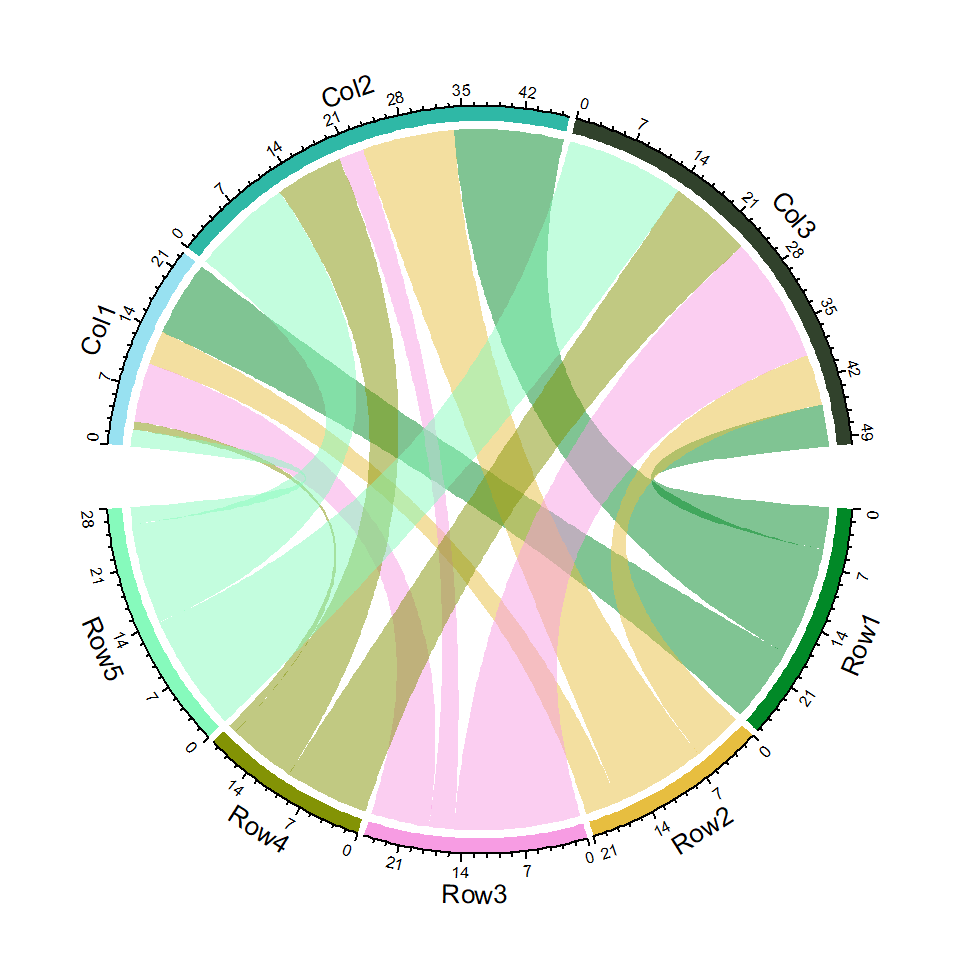## Color customization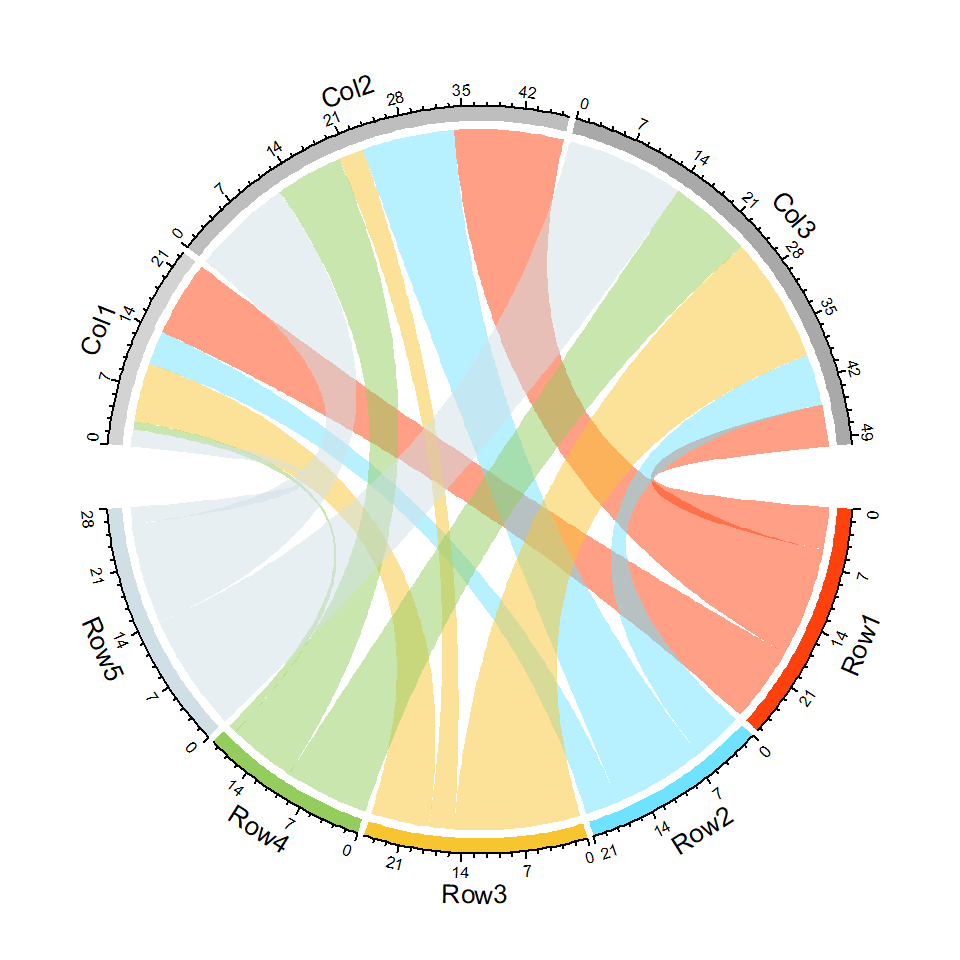Grid colors

The colors representing each columns or rows of the matrix can be customized passing a named vector to the `grid.col` argument of the function.

``````# install.packages("circlize")
library(circlize)

colors <- c(Col1 = "lightgrey", Col2 = "grey",
Col3 = "darkgrey", Row1 = "#FF410D",
Row2 = "#6EE2FF", Row3 = "#F7C530",
Row4 = "#95CC5E", Row5 = "#D0DFE6")

chordDiagram(m, grid.col = colors)
circos.clear()``````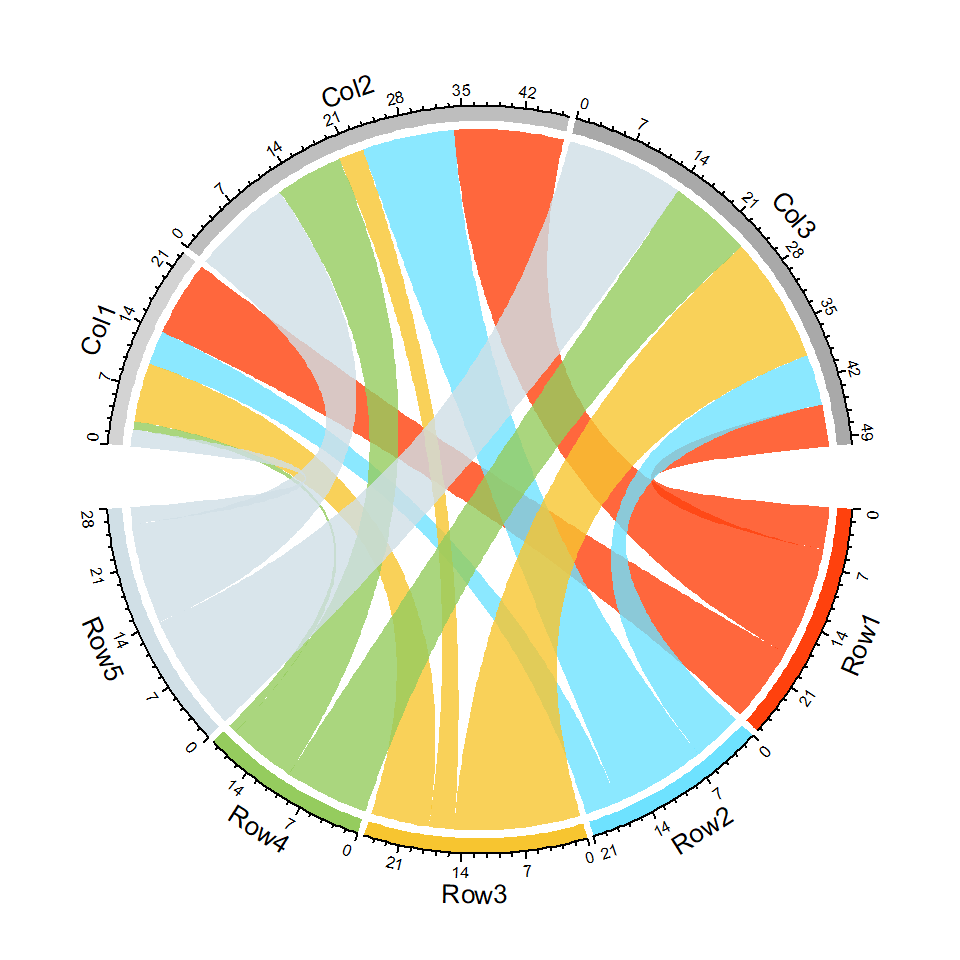Transparency

The `transparency` argument controls the level of transparency of the arcs, the lower the more opaque the color. The default value is 0.5.

``````# install.packages("circlize")
library(circlize)

colors <- c(Col1 = "lightgrey", Col2 = "grey",
Col3 = "darkgrey", Row1 = "#FF410D",
Row2 = "#6EE2FF", Row3 = "#F7C530",
Row4 = "#95CC5E", Row5 = "#D0DFE6")

chordDiagram(m, grid.col = colors,
transparency = 0.2)
circos.clear()``````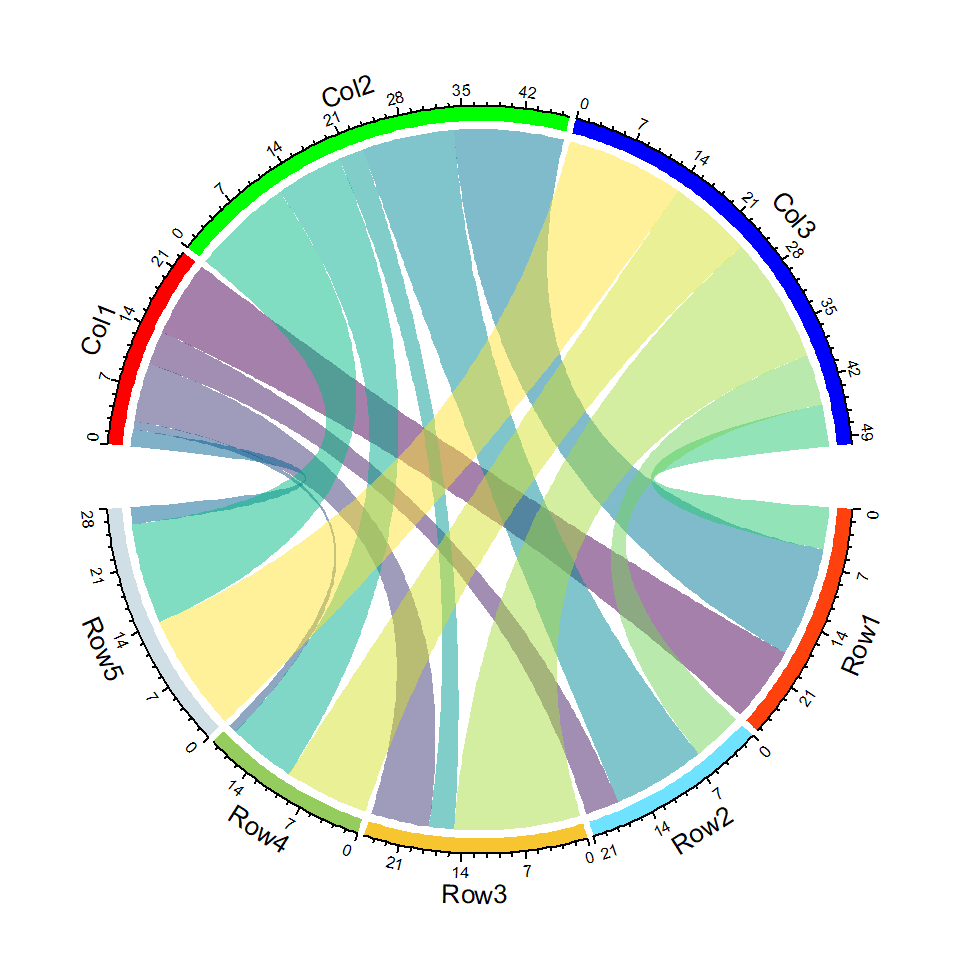The colors of the links can be controlled with the `col` argument. You will need to specify as many colors as the number of elements of the matrix. Alternatively you can use `row.col` and `column.col`.

``````# install.packages("circlize")
library(circlize)

colors <- c(Col1 = "red", Col2 = "green",
Col3 = "blue", Row1 = "#FF410D",
Row2 = "#6EE2FF", Row3 = "#F7C530",
Row4 = "#95CC5E", Row5 = "#D0DFE6")

chordDiagram(m, grid.col = colors,
col = hcl.colors(15))
circos.clear()``````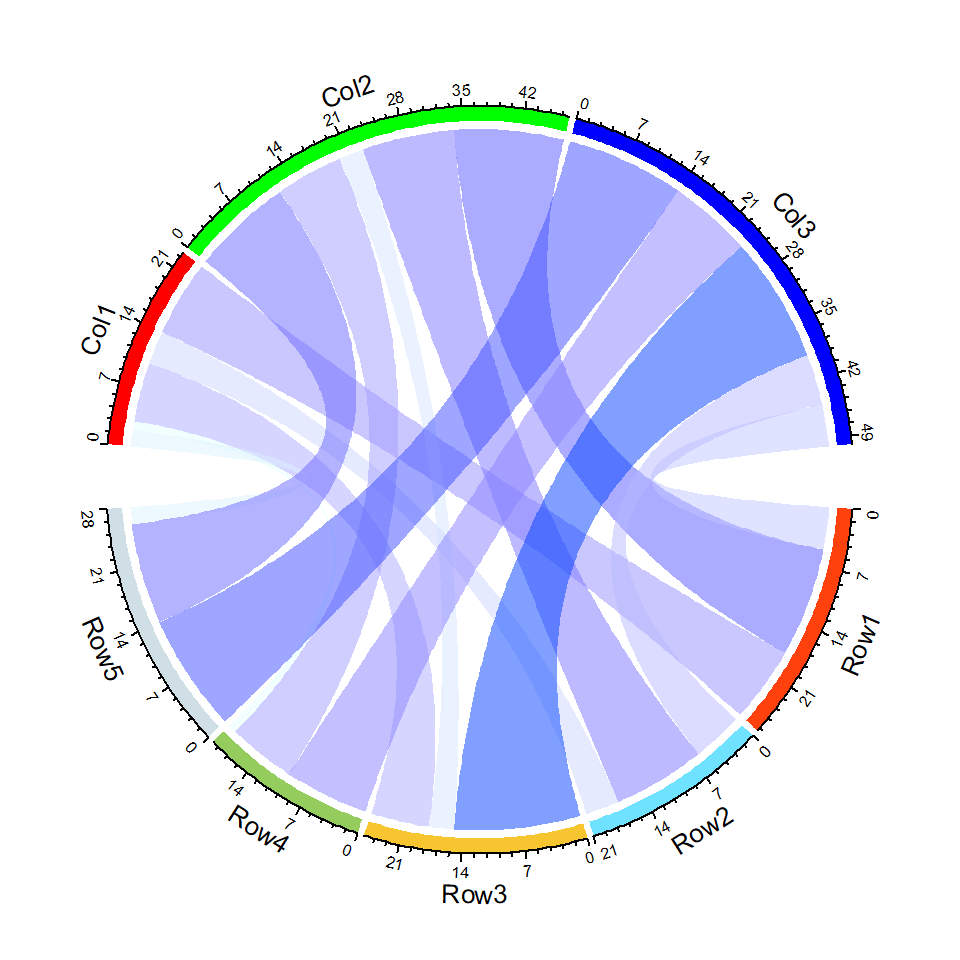Using a color ramp palette

Note that the `col` argument also admits a color function created with `colorRamp2`, as shown in the example below.

``````# install.packages("circlize")
library(circlize)

colors <- c(Col1 = "red", Col2 = "green",
Col3 = "blue", Row1 = "#FF410D",
Row2 = "#6EE2FF", Row3 = "#F7C530",
Row4 = "#95CC5E", Row5 = "#D0DFE6")
cols <- colorRamp2(range(m), c("#E5FFFF", "#003FFF"))

chordDiagram(m, grid.col = colors, col = cols)
circos.clear()``````

The border of the links can be customized with `link.lwd`, `link.lty` and `link.border` for width, type and color of the link lines.

``````# install.packages("circlize")
library(circlize)

cols <- hcl.colors(15, "Temps")

chordDiagram(m,
col = cols,
transparency = 0.1,
link.lwd = 1,    # Line width
link.lty = 1,    # Line type
link.border = 1) # Border color

circos.clear()``````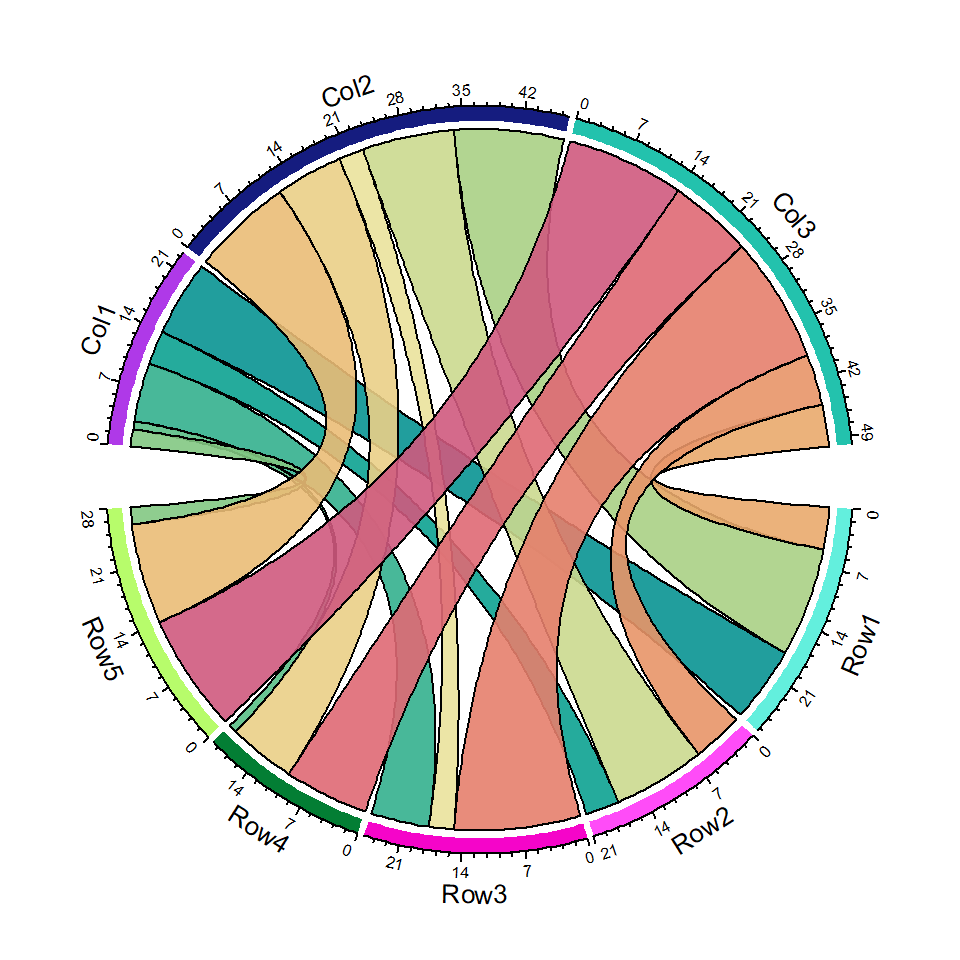Border of specific rows

It should be noted that the `link.border` argument accepts a matrix as input. In the following example we are highlighting the third row of the matrix.

``````# install.packages("circlize")
library(circlize)

cols <- hcl.colors(15, "Geyser")
mb <- matrix("black", nrow = 1, ncol = ncol(m))
rownames(mb) <- rownames(m) # Third row
colnames(mb) <- colnames(m)

chordDiagram(m,
col = cols,
transparency = 0.1,
link.lwd = 2,     # Line width
link.lty = 2,     # Line type
link.border = mb) # Border color

circos.clear()``````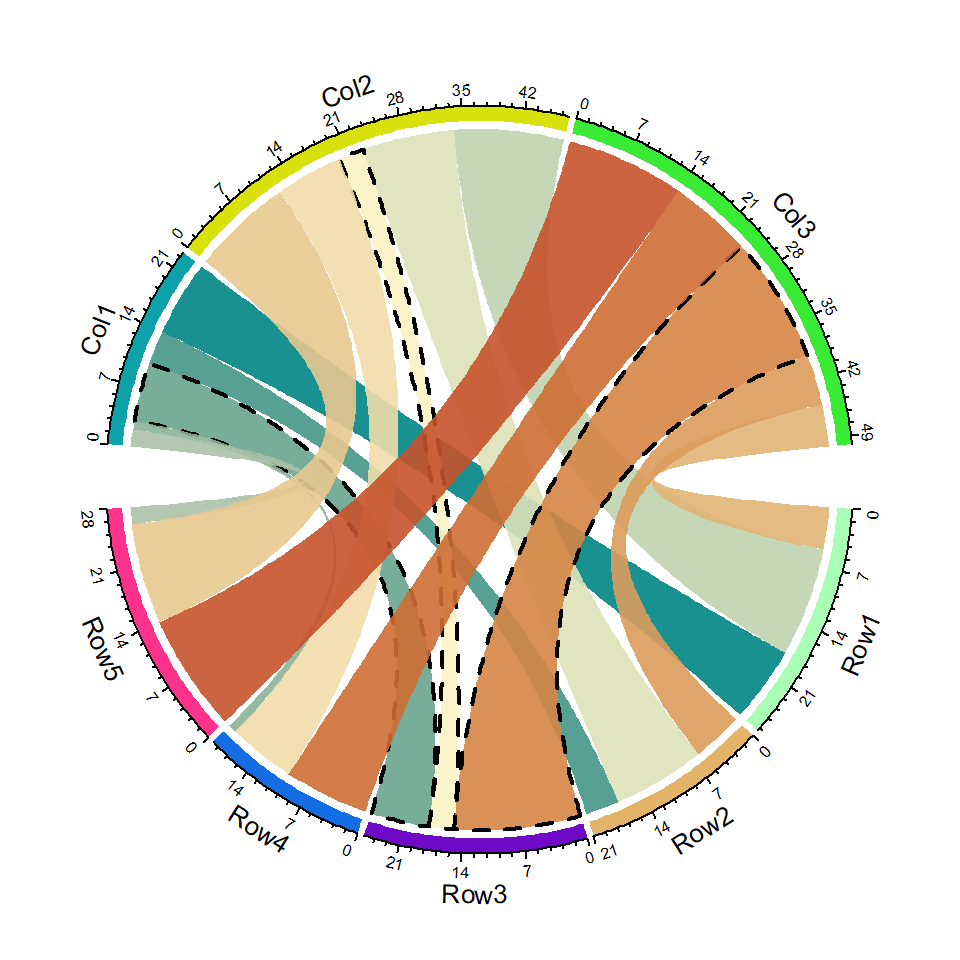## Diagram tracks

By default, the function draws a track with sector names and a track containing the grid and the axis.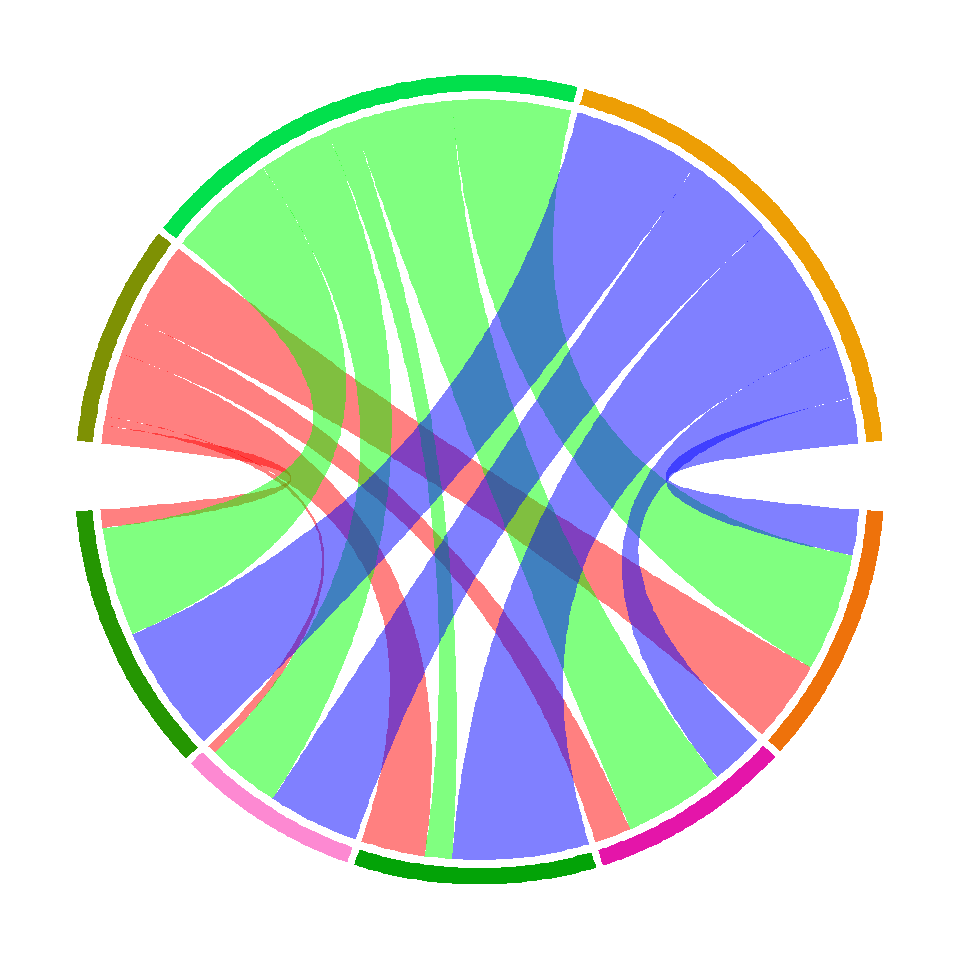Grid track

Setting `annotationTrack = "grid"` will add only the grid. The height of the track can be customized with `annotationTrackHeight`.

``````# install.packages("circlize")
library(circlize)

chordDiagram(m, column.col = c("red", "green", "blue"),
annotationTrack = "grid",
annotationTrackHeight = c(0.04, 0.02))

circos.clear()``````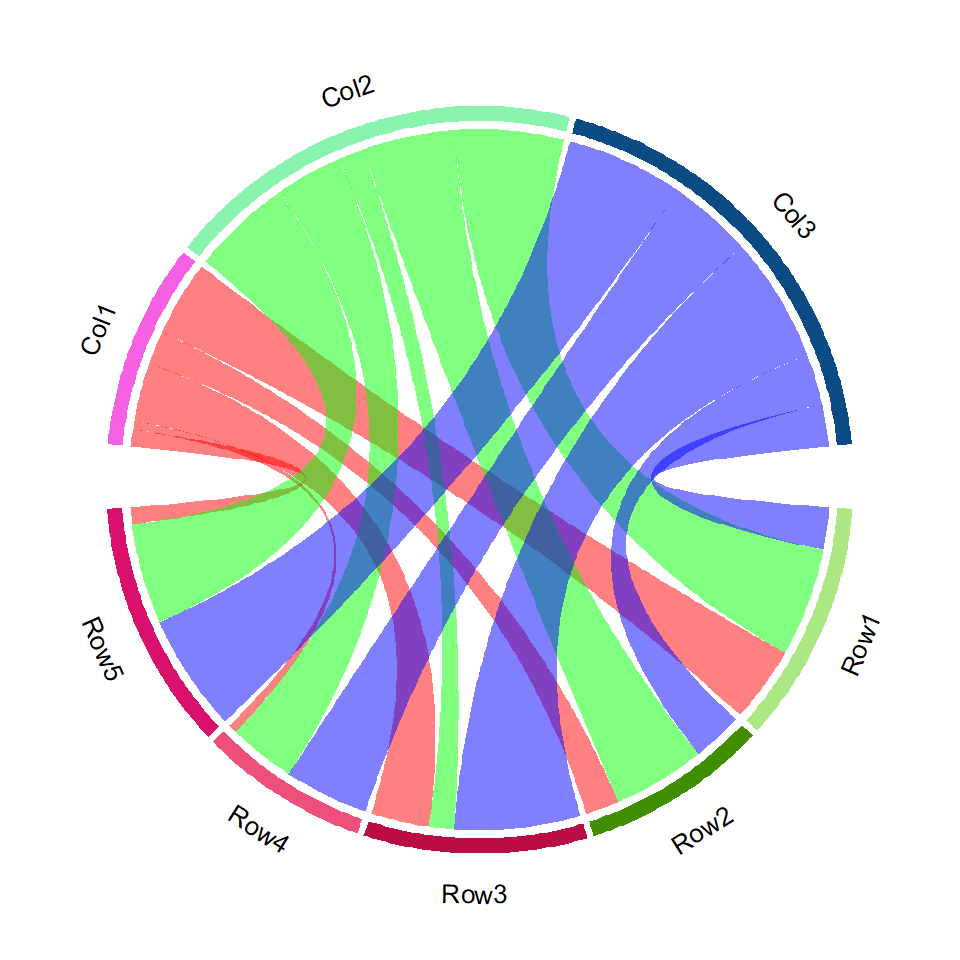Names and grid track

You can also draw the names and the grid but not the axis.

``````# install.packages("circlize")
library(circlize)

chordDiagram(m, column.col = c("red", "green", "blue"),
annotationTrack =  c("name", "grid"))

circos.clear()``````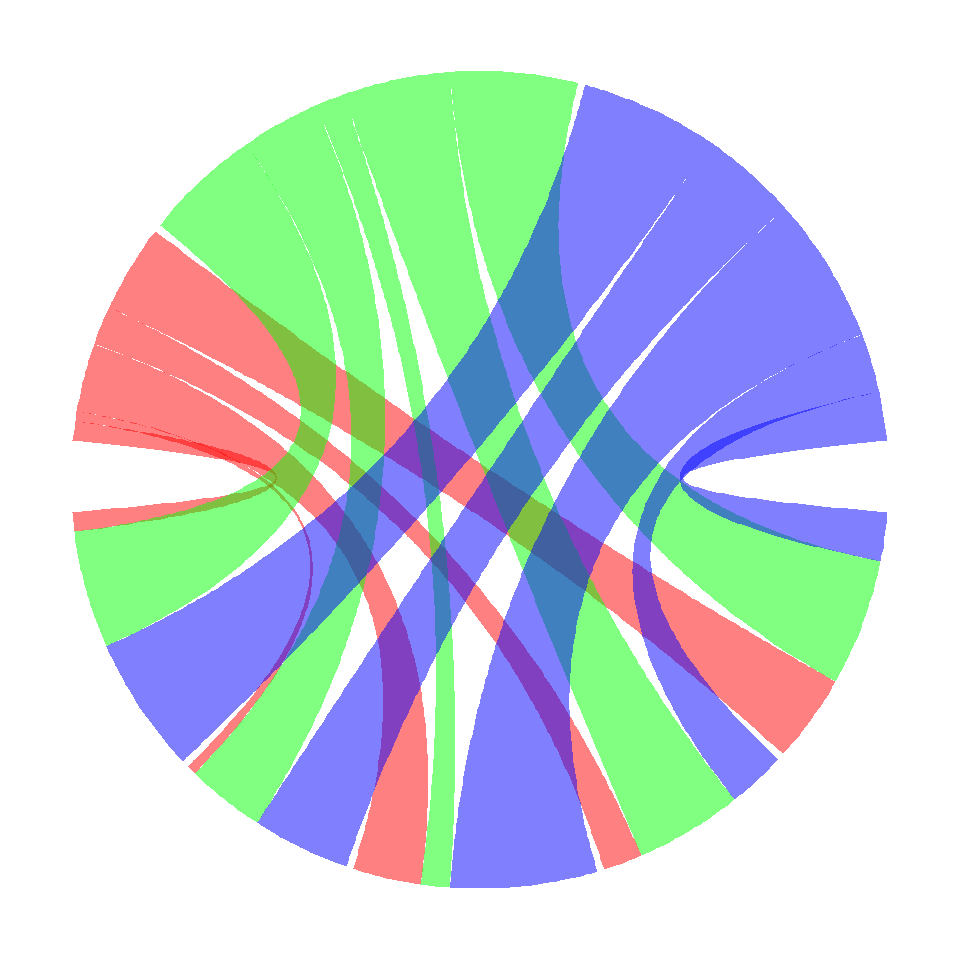No track

Finally, if you want to remove the track set the `annotationTrack` argument to `NULL`.

``````# install.packages("circlize")
library(circlize)

chordDiagram(m, column.col = c("red", "green", "blue"),
annotationTrack = NULL)

circos.clear()``````